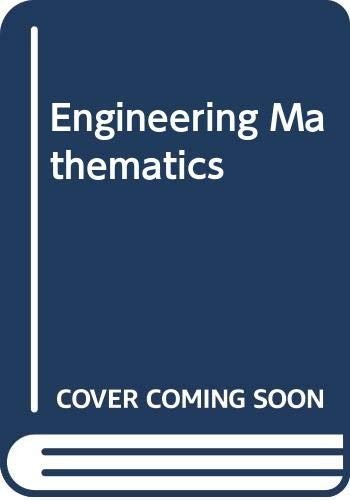# Engineering Mathematics by John O. Bird

In Stock
\$28.99
inc. GST
The aim of this text is to develop the undergraduate student's use of mathematics appropriate for entry to an engineering degree course. Illustrated with exercises and worked problems, the book has been devised primarily as an option in courses leading to a BTEC National Diploma in Engineering.
Only 3 left

## Engineering Mathematics Summary

### Engineering Mathematics by John O. Bird

Unfortunately we do not have a summary for this item at the moment

### Why buy from World of BooksOur excellent value books literally don't cost the earthFree delivery in AustraliaEvery used book bought is one saved from landfill

Exponential functions and Napierian logarithms; complex numbers - Cartesian complex numbers, the Argand diagram, De Moivre's theorem; polar co-ordinates; partial fractions; the binomial theorem - Pascal's triangle; arithmetical and geometric progressions; the theory of matrices and determinants; the solution of simultaneous equations by matrices and determinants; the solution of triangles and their areas; the solution of three-dimensional triangulation problems; graphs of sine and cosine functions; combining periodic waveforms; compound angles; vectors, vector addition and subtraction and vector products; differentiation from first principles; methods of differentiation; applications of differentiation; introduction to integration; integration using substitutions and partial fractions; integration by parts; numerical integration - Simpson's rule; areas under and between curves; mean and root mean square values; volumes of solids of revolution; centroids of simple shapes - theorem of Pappus; second moments of areas of regular sections; introduction to differential equations; solution of first order differential equations by separation of variables.

GOR003330572
Engineering Mathematics by John O. Bird
John O. Bird
Used - Very Good
Paperback
Elsevier Science & Technology
1989-04-03
336
0434901229
9780434901227
N/A
Book picture is for illustrative purposes only, actual binding, cover or edition may vary.
This is a used book - there is no escaping the fact it has been read by someone else and it will show signs of wear and previous use. Overall we expect it to be in very good condition, but if you are not entirely satisfied please get in touch with us.